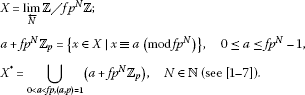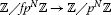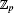Theory and Modern Applications

Frobenius-Euler polynomials and umbral calculus in the p-adic case

Abstract

In this paper, we study some p-adic Frobenius-Euler measure related to umbral calculus in the p-adic case. Finally, we derive some identities of Frobenius-Euler polynomials from our study.

MSC:05A10, 05A19.

1 Introduction

Let p be a fixed prime number. Throughout this paper ${\mathbb{Z}}_{p}$, ${\mathbb{Q}}_{p}$ and ${\mathbb{C}}_{p}$ will denote the ring of p-adic integers, the field of p-adic rational numbers and the completion of algebraic closure of ${\mathbb{Q}}_{p}$, respectively.

For $f\in \mathbb{N}$ with $\left(f,p\right)=1$, letNote that the natural mapinduces

$\pi :X\to {\mathbb{Z}}_{p}.$

If g is a function on ${\mathbb{Z}}_{p}$, we denote by the same g the function $g\circ \pi$ on X. Namely, we can consider g as a function on X.

For $k\ge 0$ and $\lambda \in {\mathbb{C}}_{p}$ with ${|1-\lambda |}_{p}>1$, the Frobenius-Euler measure on X is defined by

${\mu }_{\lambda }\left(x+f{p}^{N}{\mathbb{Z}}_{p}\right)=\frac{{\lambda }^{f{p}^{N}-x}}{1-{\lambda }^{f{p}^{N}}}\phantom{\rule{1em}{0ex}}\left(\text{see [5, 8])},$
(1.1)

where the p-adic absolute value on ${\mathbb{C}}_{p}$ is normalized by ${|p|}_{p}=\frac{1}{p}$.

As is well known, the Frobenius-Euler polynomials are defined by the generating function to be

$\left(\frac{1-\lambda }{{e}^{t}-\lambda }\right){e}^{xt}={e}^{H\left(x|\lambda \right)t}=\sum _{n=0}^{\mathrm{\infty }}{H}_{n}\left(x|\lambda \right)\frac{{t}^{n}}{n!}\phantom{\rule{1em}{0ex}}\left(\text{see [5, 7, 9])},$
(1.2)

with the usual convention about replacing ${H}^{n}\left(x|\lambda \right)$ by ${H}_{n}\left(x|\lambda \right)$. In the special case, $x=0$, ${H}_{n}\left(0|\lambda \right)={H}_{n}\left(\lambda \right)$ are called the n th Frobenius-Euler numbers

${H}_{n}\left(x|\lambda \right)={\left(H\left(\lambda \right)+x\right)}^{n}=\sum _{l=0}^{\mathrm{\infty }}\left(\genfrac{}{}{0}{}{n}{l}\right){H}_{l}\left(\lambda \right){x}^{n-l}\phantom{\rule{1em}{0ex}}\text{(see [6, 9, 10])}.$
(1.3)

Thus, by (1.2) and (1.3), we easily get

${\left(H\left(\lambda \right)+1\right)}^{n}-\lambda {H}_{n}\left(\lambda \right)=\left(1-\lambda \right){\delta }_{0,n}\phantom{\rule{1em}{0ex}}\left(\text{see [1–19])},$
(1.4)

where ${\delta }_{n,k}$ is the Kronecker symbol.

For $r\in \mathbb{N}$, the Frobenius-Euler polynomials of order r are defined by the generating function

$\begin{array}{rl}{\left(\frac{1-\lambda }{{e}^{t}-\lambda }\right)}^{r}{e}^{xt}& =\underset{r\text{-times}}{\underset{⏟}{\left(\frac{1-\lambda }{{e}^{t}-\lambda }\right)×\cdots ×\left(\frac{1-\lambda }{{e}^{t}-\lambda }\right)}}{e}^{xt}\\ =\sum _{n=0}^{\mathrm{\infty }}{H}_{n}^{\left(r\right)}\left(x|\lambda \right)\frac{{t}^{n}}{n!}\phantom{\rule{1em}{0ex}}\left(\text{see [5, 9])}.\end{array}$
(1.5)

In the special case, $x=0$, ${H}_{n}^{\left(r\right)}\left(0|\lambda \right)={H}_{n}^{\left(r\right)}\left(\lambda \right)$ are called the n th Frobenius-Euler numbers of order r. The n th Frobenius-Euler polynomials can be represented by (1.1) as follows:

$\begin{array}{rl}\frac{\lambda {H}_{n}\left(x|\lambda \right)}{1-\lambda }& ={\int }_{X}{\left(x+y\right)}^{n}\phantom{\rule{0.2em}{0ex}}d{\mu }_{\lambda }\left(y\right)={\int }_{{\mathbb{Z}}_{p}}{\left(x+y\right)}^{n}\phantom{\rule{0.2em}{0ex}}d{\mu }_{\lambda }\left(y\right)\\ =\underset{N\to \mathrm{\infty }}{lim}\frac{1}{1-{\lambda }^{{p}^{N}}}\sum _{y=0}^{{p}^{N}-1}{\left(x+y\right)}^{n}{\lambda }^{{p}^{N}-y}\phantom{\rule{1em}{0ex}}\left(\text{see [6, 7])}.\end{array}$
(1.6)

Let be the set of all formal power series in the variable t over ${\mathbb{C}}_{p}$ with

$\mathcal{F}=\left\{f\left(t\right)=\sum _{k=0}^{\mathrm{\infty }}\frac{{a}_{k}}{k!}{t}^{k}|{a}_{k}\in {\mathbb{C}}_{p}\right\}.$
(1.7)

Let $\mathbb{P}={\mathbb{C}}_{p}\left[x\right]$ and ${\mathbb{P}}^{\ast }$ denote the vector space of all linear functionals on .

The formal power series

$f\left(t\right)=\sum _{k=0}^{\mathrm{\infty }}\frac{{a}_{k}}{k!}{t}^{k}\in \mathcal{F}\phantom{\rule{1em}{0ex}}\left(\text{see [11, 15])}$
(1.8)

defines a linear functional on by setting

(1.9)

From (1.8) and (1.9), we have

$〈{t}^{n}|{x}^{n}〉=n!{\delta }_{n,k}\phantom{\rule{1em}{0ex}}\left(n,k\ge 0\right).$
(1.10)

Here, denotes both the algebra of formal power series in t and the vector space of all linear functionals on , and so an element $f\left(t\right)$ of will be thought of as both a formal power series and a linear functional (see [11, 15]). We will call the umbral algebra. The umbral calculus is the study of umbral algebra (see [11, 15]).

The order $o\left(f\left(t\right)\right)$ of power series $f\left(t\right)$ (≠0) is the smallest integer k for which ${a}_{k}$ does not vanish (see [11, 15]). A series $f\left(t\right)$ for which $o\left(f\left(t\right)\right)=1$ is called a delta series. If a series $f\left(t\right)$ has $o\left(f\left(t\right)\right)=0$, then $f\left(t\right)$ is called an invertible series (see [11, 15]). Let $f\left(t\right),g\left(t\right)\in \mathcal{F}$. Then we easily see that $〈f\left(t\right)g\left(t\right)|p\left(x\right)〉=〈f\left(t\right)|g\left(t\right)p\left(x\right)〉=〈g\left(t\right)|f\left(t\right)p\left(x\right)〉$. From (1.10), we note that

$〈{e}^{yt}|{x}^{n}〉={y}^{n},\phantom{\rule{2em}{0ex}}〈{e}^{yt}|p\left(x\right)〉=p\left(y\right),$
(1.11)
$f\left(t\right)=\sum _{k=0}^{\mathrm{\infty }}\frac{〈f\left(t\right)|{x}^{k}〉}{k!}{t}^{k},\phantom{\rule{1em}{0ex}}f\left(t\right)\in \mathcal{F},$
(1.12)

and

(1.13)

For ${f}_{1}\left(t\right),{f}_{2}\left(t\right),\dots ,{f}_{m}\left(t\right)\in \mathcal{F}$, we have

$〈{f}_{1}\left(t\right){f}_{2}\left(t\right)\cdots {f}_{m}\left(t\right)|{x}^{n}〉=\sum _{{i}_{1}+\cdots +{i}_{m}=n}\left(\genfrac{}{}{0}{}{n}{{i}_{1},\dots ,{i}_{m}}\right)〈{f}_{1}\left(t\right)|{x}^{{i}_{1}}〉\cdots 〈{f}_{m}\left(t\right)|{x}^{{i}_{m}}〉.$
(1.14)

By (1.13), we get

${p}^{\left(k\right)}\left(x\right)=\frac{{d}^{k}p\left(x\right)}{d{x}^{k}}=\sum _{l=k}^{n}〈{t}^{l}|p\left(x\right)〉\left(\genfrac{}{}{0}{}{l}{k}\right)\frac{k!}{l!}{x}^{l-k}$
(1.15)

and

${p}^{\left(k\right)}\left(0\right)=〈{t}^{k}|p\left(x\right)〉=〈1|{p}^{\left(k\right)}\left(x\right)〉.$

Thus, by (1.15), we get

${t}^{k}p\left(x\right)={p}^{\left(k\right)}\left(x\right)=\frac{{d}^{k}p\left(x\right)}{d{x}^{k}}\phantom{\rule{1em}{0ex}}\text{(see [11, 15])}.$
(1.16)

By (1.16), we easily see that

${e}^{yt}p\left(x\right)=p\left(x+y\right)\phantom{\rule{1em}{0ex}}\text{(see )}.$
(1.17)

Let ${S}_{n}\left(x\right)$ denote a polynomial of degree n. Suppose that $f\left(t\right),g\left(t\right)\in \mathcal{F}$ with $o\left(f\left(t\right)\right)=1$ and $o\left(g\left(t\right)\right)=0$. Then there exists a unique sequence ${S}_{n}\left(x\right)$ of polynomials satisfying $〈g\left(t\right)f{\left(t\right)}^{k}|{S}_{n}\left(x\right)〉=n!{\delta }_{n,k}$ for all $n,k\ge 0$. The sequence ${S}_{n}\left(x\right)$ is called the Sheffer sequence for $\left(g\left(t\right),f\left(t\right)\right)$, which is denoted by ${S}_{n}\left(x\right)\sim \left(g\left(t\right),f\left(t\right)\right)$. If ${S}_{n}\left(x\right)\sim \left(g\left(t\right),t\right)$, then ${S}_{n}\left(x\right)$ is called the Appell sequence for $g\left(t\right)$ (see ).

For $p\left(x\right)\in \mathbb{P}$, we have

$〈f\left(t\right)|xp\left(x\right)〉=〈{\partial }_{t}f\left(t\right)|p\left(x\right)〉=〈{f}^{\mathrm{\prime }}\left(t\right)|p\left(x\right)〉,$
(1.18)
$〈{e}^{yt}-1|p\left(x\right)〉=p\left(y\right)-p\left(0\right).$
(1.19)

If ${S}_{n}\left(x\right)\sim \left(g\left(t\right),f\left(t\right)\right)$, then we have

$h\left(t\right)=\sum _{k=0}^{\mathrm{\infty }}\frac{〈h\left(t\right)|{S}_{k}\left(x\right)〉}{k!}g\left(t\right)f{\left(t\right)}^{k},\phantom{\rule{1em}{0ex}}h\left(t\right)\in \mathcal{F},$
(1.20)
$p\left(x\right)=\sum _{k=0}^{\mathrm{\infty }}\frac{〈g\left(t\right)f{\left(t\right)}^{k}|p\left(x\right)〉}{k!}{S}_{k}\left(x\right),\phantom{\rule{1em}{0ex}}p\left(x\right)\in \mathbb{P},$
(1.21)
$f\left(t\right){S}_{n}\left(x\right)=n{S}_{n-1}\left(x\right),$
(1.22)

and

(1.23)

where $\overline{f}\left(t\right)$ is compositional inverse of $f\left(t\right)$ (see [11, 15]). In , Kim and Kim have studied some identities of Frobenius-Euler polynomials arising from umbral calculus. In this paper, we study some p-adic Frobenius-Euler integral onrelated to umbral calculus in the p-adic case. Finally, we derive some new and interesting identities of Frobenius-Euler polynomials from our study.

2 Frobenius-Euler polynomials associated with umbral calculus

Let

$g\left(t;\lambda \right)=\frac{{e}^{t}-\lambda }{1-\lambda }\in \mathcal{F}.$
(2.1)

Then we see that $g\left(t;\lambda \right)$ is an invertible series. From (1.2), we have

$\sum _{k=0}^{\mathrm{\infty }}{H}_{k}\left(x|\lambda \right)\frac{{t}^{k}}{k!}=\frac{1}{g\left(t;\lambda \right)}{e}^{xt}.$
(2.2)

Hence, by (2.2), we get

$\left(\frac{1-\lambda }{{e}^{t}-\lambda }\right){x}^{n}=\frac{1}{g\left(t;\lambda \right)}{x}^{n}={H}_{n}\left(x|\lambda \right).$
(2.3)

By (2.2) and (2.3), we get

${H}_{n}\left(x|\lambda \right)\sim \left(g\left(t;\lambda \right),t\right).$

From (1.6), we have

${\int }_{{\mathbb{Z}}_{p}}{e}^{\left(x+y\right)t}\phantom{\rule{0.2em}{0ex}}d{\mu }_{\lambda }\left(y\right)=\frac{\lambda }{{e}^{t}-\lambda }{e}^{xt},$
(2.4)

and

${\int }_{{\mathbb{Z}}_{p}}{e}^{\left(x+y+1\right)t}\phantom{\rule{0.2em}{0ex}}d{\mu }_{\lambda }\left(y\right)-\lambda {\int }_{{\mathbb{Z}}_{p}}{e}^{\left(x+y\right)t}\phantom{\rule{0.2em}{0ex}}d{\mu }_{\lambda }\left(y\right)=\lambda {e}^{xt}.$
(2.5)

By (2.5), we get

${\int }_{{\mathbb{Z}}_{p}}{\left(x+y+1\right)}^{n}\phantom{\rule{0.2em}{0ex}}d{\mu }_{\lambda }\left(y\right)-\lambda {\int }_{{\mathbb{Z}}_{p}}{\left(x+y\right)}^{n}\phantom{\rule{0.2em}{0ex}}d{\mu }_{\lambda }\left(y\right)=\lambda {x}^{n}.$
(2.6)

From (1.6) and (2.6), we have

$\frac{\lambda }{1-\lambda }{H}_{n}\left(x+1|\lambda \right)-\frac{{\lambda }^{2}}{1-\lambda }{H}_{n}\left(x|\lambda \right)=\lambda {x}^{n}.$
(2.7)

From (2.2), we can easily derive

${H}_{n+1}\left(x|\lambda \right)=\left(x-\frac{{g}^{\prime }\left(t;\lambda \right)}{g\left(t;\lambda \right)}\right){H}_{n}\left(x|\lambda \right).$
(2.8)

By (2.8), we get

$g\left(t;\lambda \right){H}_{n+1}\left(x|\lambda \right)=g\left(t;\lambda \right)x{H}_{n}\left(x|\lambda \right)-{g}^{\prime }\left(t;\lambda \right){H}_{n}\left(x|\lambda \right).$
(2.9)

Thus, from (2.9), we have

$\left({e}^{t}-\lambda \right){H}_{n+1}\left(x|\lambda \right)=\left({e}^{t}-\lambda \right)x{H}_{n}\left(x|\lambda \right)-{e}^{t}{H}_{n}\left(x|\lambda \right).$
(2.10)

By (2.10), we get

${H}_{n+1}\left(x+1|\lambda \right)-\lambda {H}_{n+1}\left(x|\lambda \right)=x\left({H}_{n}\left(x+1|\lambda \right)-\lambda {H}_{n}\left(x|\lambda \right)\right).$
(2.11)

From (2.11), we note that

$\begin{array}{rl}{H}_{n}\left(x+1|\lambda \right)-\lambda {H}_{n}\left(x|\lambda \right)& =x\left({H}_{n-1}\left(x+1|\lambda \right)-\lambda {H}_{n-1}\left(x|\lambda \right)\right)\\ ={x}^{2}\left({H}_{n-2}\left(x+1|\lambda \right)-\lambda {H}_{n-2}\left(x|\lambda \right)\right)=\cdots \\ ={x}^{n}\left({H}_{0}\left(x+1|\lambda \right)-\lambda {H}_{0}\left(x|\lambda \right)\right)={x}^{n}\left(1-\lambda \right).\end{array}$
(2.12)

Let us consider the linear functional $f\left(t\right)$ such that

$〈f\left(t\right)|p\left(x\right)〉={\int }_{{\mathbb{Z}}_{p}}p\left(u\right)\phantom{\rule{0.2em}{0ex}}d{\mu }_{\lambda }\left(u\right)$
(2.13)

for all polynomials $p\left(x\right)$ can be determined from (1.12) to be

$f\left(t\right)=\sum _{k=0}^{\mathrm{\infty }}\frac{〈f\left(t\right)|{x}^{k}〉}{k!}{t}^{k}=\sum _{k=0}^{\mathrm{\infty }}{\int }_{{\mathbb{Z}}_{p}}{u}^{k}\phantom{\rule{0.2em}{0ex}}d{\mu }_{\lambda }\left(u\right)\frac{{t}^{k}}{k!}={\int }_{{\mathbb{Z}}_{p}}{e}^{ut}\phantom{\rule{0.2em}{0ex}}d{\mu }_{\lambda }\left(u\right).$
(2.14)

By (2.4) and (2.14), we get

$f\left(t\right)={\int }_{{\mathbb{Z}}_{p}}{e}^{ut}\phantom{\rule{0.2em}{0ex}}d{\mu }_{\lambda }\left(u\right)=\frac{\lambda }{{e}^{t}-\lambda }.$
(2.15)

Therefore, by (2.15), we obtain the following theorem.

Theorem 2.1 For $p\left(x\right)\in \mathbb{P}$, we have

$〈\frac{\lambda }{{e}^{t}-\lambda }|p\left(x\right)〉={\int }_{{\mathbb{Z}}_{p}}p\left(u\right)\phantom{\rule{0.2em}{0ex}}d{\mu }_{\lambda }\left(u\right).$

In particular,

$\frac{\lambda }{1-\lambda }{H}_{n}\left(\lambda \right)=〈{\int }_{{\mathbb{Z}}_{p}}{e}^{yt}\phantom{\rule{0.2em}{0ex}}d{\mu }_{\lambda }\left(y\right)|{x}^{n}〉.$

From (1.6), we have

$\sum _{n=0}^{\mathrm{\infty }}{\int }_{{\mathbb{Z}}_{p}}{\left(x+y\right)}^{n}\phantom{\rule{0.2em}{0ex}}d{\mu }_{\lambda }\left(y\right)\frac{{t}^{n}}{n!}={\int }_{{\mathbb{Z}}_{p}}{e}^{\left(x+y\right)t}\phantom{\rule{0.2em}{0ex}}d{\mu }_{\lambda }\left(y\right)=\sum _{n=0}^{\mathrm{\infty }}{\int }_{{\mathbb{Z}}_{p}}{e}^{yt}\phantom{\rule{0.2em}{0ex}}d{\mu }_{\lambda }\left(y\right){x}^{n}\frac{{t}^{n}}{n!}.$
(2.16)

By (1.6), (2.4) and (2.16), we get

(2.17)

Therefore, by (2.17), we obtain the following theorem.

Theorem 2.2 For $p\left(x\right)\in \mathbb{P}$, we have

${\int }_{{\mathbb{Z}}_{p}}p\left(x+y\right)\phantom{\rule{0.2em}{0ex}}d{\mu }_{\lambda }\left(y\right)={\int }_{{\mathbb{Z}}_{p}}{e}^{yt}\phantom{\rule{0.2em}{0ex}}d{\mu }_{\lambda }\left(y\right)p\left(x\right)=\frac{\lambda }{{e}^{t}-\lambda }p\left(x\right).$

In particular,

$\frac{\lambda }{1-\lambda }{H}_{n}\left(x|\lambda \right)={\int }_{{\mathbb{Z}}_{p}}{e}^{yt}\phantom{\rule{0.2em}{0ex}}d{\mu }_{\lambda }\left(y\right){x}^{n}=\frac{\lambda }{{e}^{t}-\lambda }{x}^{n}\phantom{\rule{1em}{0ex}}\left(n\ge 0\right).$

By (1.6) and (2.16), we get

$\frac{\lambda }{1-\lambda }{H}_{n}\left(x|\lambda \right)\sim \left(\frac{{e}^{t}-\lambda }{\lambda },t\right).$
(2.18)

From Appell identity and (2.18), we can derive the following identities:

${H}_{n}\left(x+y|\lambda \right)=\sum _{k=0}^{n}\left(\genfrac{}{}{0}{}{n}{k}\right){H}_{k}\left(x|\lambda \right){y}^{n-k}.$
(2.19)

Let

${g}^{r}\left(t;\lambda \right)={\left(\frac{{e}^{t}-\lambda }{\lambda }\right)}^{r}=\underset{r\text{-times}}{\underset{⏟}{\left(\frac{{e}^{t}-\lambda }{\lambda }\right)×\cdots ×\left(\frac{{e}^{t}-\lambda }{\lambda }\right)}}\in \mathcal{F}.$
(2.20)

Then ${g}^{r}\left(t;\lambda \right)$ is an invertible functional in . By (1.5) and (2.20), we get

$\frac{1}{{g}^{r}\left(t;\lambda \right)}{e}^{xt}=\frac{{\lambda }^{r}}{{\left(1-\lambda \right)}^{r}}\sum _{k=0}^{\mathrm{\infty }}{H}_{n}^{\left(r\right)}\left(x|\lambda \right)\frac{{t}^{n}}{n!}.$
(2.21)

Thus, from (2.21), we have

$\frac{1}{{g}^{r}\left(t;\lambda \right)}{x}^{n}={\left(\frac{\lambda }{1-\lambda }\right)}^{r}{H}_{n}^{\left(r\right)}\left(x|\lambda \right),$
(2.22)

and

${\left(\frac{\lambda }{1-\lambda }\right)}^{r}t{H}_{n}^{\left(r\right)}\left(x|\lambda \right)=\frac{n}{{g}^{r}\left(t;\lambda \right)}{x}^{n-1}=n{\left(\frac{\lambda }{1-\lambda }\right)}^{r}{H}_{n-1}^{\left(r\right)}\left(x|\lambda \right).$
(2.23)

By (2.22) and (2.23), we see that

${\left(\frac{\lambda }{1-\lambda }\right)}^{r}{H}_{n}^{\left(r\right)}\left(x|\lambda \right)\sim \left({g}^{r}\left(t;\lambda \right),t\right).$
(2.24)

From (2.4), we can derive the following identity:

$\begin{array}{r}\underset{r\text{-times}}{\underset{⏟}{{\int }_{{\mathbb{Z}}_{p}}\cdots {\int }_{{\mathbb{Z}}_{p}}}}{e}^{\left({x}_{1}+{x}_{2}+\cdots +{x}_{r}+x\right)t}\phantom{\rule{0.2em}{0ex}}d{\mu }_{\lambda }\left({x}_{1}\right)\cdots \phantom{\rule{0.2em}{0ex}}d{\mu }_{\lambda }\left({x}_{r}\right)\\ \phantom{\rule{1em}{0ex}}={\left(\frac{\lambda }{{e}^{t}-\lambda }\right)}^{r}{e}^{xt}={\left(\frac{\lambda }{1-\lambda }\right)}^{r}\sum _{n=0}^{\mathrm{\infty }}{H}_{n}^{\left(r\right)}\left(x|\lambda \right)\frac{{t}^{n}}{n!}.\end{array}$
(2.25)

By (1.10) and (2.25), we get

$\begin{array}{r}{\left(\frac{\lambda }{1-\lambda }\right)}^{r}{H}_{n}^{\left(r\right)}\left(x|\lambda \right)\\ \phantom{\rule{1em}{0ex}}=〈\underset{r\text{-times}}{\underset{⏟}{{\int }_{{\mathbb{Z}}_{p}}\cdots {\int }_{{\mathbb{Z}}_{p}}}}{e}^{\left({x}_{1}+{x}_{2}+\cdots +{x}_{r}+x\right)t}\phantom{\rule{0.2em}{0ex}}d{\mu }_{\lambda }\left({x}_{1}\right)\cdots \phantom{\rule{0.2em}{0ex}}d{\mu }_{\lambda }\left({x}_{r}\right)|{x}^{n}〉.\end{array}$
(2.26)

From (1.14), we have

$\begin{array}{r}〈\underset{r\text{-times}}{\underset{⏟}{{\int }_{{\mathbb{Z}}_{p}}\cdots {\int }_{{\mathbb{Z}}_{p}}}}{e}^{\left({x}_{1}+{x}_{2}+\cdots +{x}_{r}\right)t}\phantom{\rule{0.2em}{0ex}}d{\mu }_{\lambda }\left({x}_{1}\right)\cdots \phantom{\rule{0.2em}{0ex}}d{\mu }_{\lambda }\left({x}_{r}\right)|{x}^{n}〉\\ \phantom{\rule{1em}{0ex}}=\sum _{n={i}_{1}+\cdots +{i}_{r}}\left(\genfrac{}{}{0}{}{n}{{i}_{1},\dots ,{i}_{r}}\right)〈{\int }_{{\mathbb{Z}}_{p}}{e}^{{x}_{1}t}\phantom{\rule{0.2em}{0ex}}d{\mu }_{\lambda }\left({x}_{1}\right)|{x}^{{i}_{1}}〉×\cdots \\ \phantom{\rule{2em}{0ex}}×〈{\int }_{{\mathbb{Z}}_{p}}{e}^{{x}_{r}t}\phantom{\rule{0.2em}{0ex}}d{\mu }_{\lambda }\left({x}_{r}\right)|{x}^{{i}_{r}}〉\\ \phantom{\rule{1em}{0ex}}=\sum _{n={i}_{1}+\cdots +{i}_{r}}\left(\genfrac{}{}{0}{}{n}{{i}_{1},\dots ,{i}_{r}}\right){\left(\frac{\lambda }{1-\lambda }\right)}^{r}{H}_{{i}_{1}}\left(x|\lambda \right)\cdots {H}_{{i}_{r}}\left(x|\lambda \right).\end{array}$
(2.27)

By (2.26) and (2.27), we get

${H}_{n}^{\left(r\right)}\left(x|\lambda \right)=\sum _{n={i}_{1}+\cdots +{i}_{r}}\left(\genfrac{}{}{0}{}{n}{{i}_{1},\dots ,{i}_{r}}\right){H}_{{i}_{1}}\left(x|\lambda \right)\cdots {H}_{{i}_{r}}\left(x|\lambda \right),$

where $\left(\genfrac{}{}{0}{}{n}{{i}_{1},\dots ,{i}_{r}}\right)=\frac{n!}{{i}_{1}!\cdots {i}_{r}!}$. From (2.25), we note that

${g}^{r}\left(t;\lambda \right)=\frac{1}{\underset{r\text{-times}}{\underset{⏟}{{\int }_{{\mathbb{Z}}_{p}}\cdots {\int }_{{\mathbb{Z}}_{p}}}}{e}^{\left({x}_{1}+{x}_{2}+\cdots +{x}_{r}\right)t}\phantom{\rule{0.2em}{0ex}}d{\mu }_{\lambda }\left({x}_{1}\right)\cdots \phantom{\rule{0.2em}{0ex}}d{\mu }_{\lambda }\left({x}_{r}\right)}={\left(\frac{{e}^{t}-\lambda }{\lambda }\right)}^{r}.$
(2.28)

Thus, by (2.28), we get

$\begin{array}{rl}\frac{1}{{g}^{r}\left(t;\lambda \right)}{e}^{xt}& ={\int }_{{\mathbb{Z}}_{p}}\cdots {\int }_{{\mathbb{Z}}_{p}}{e}^{\left({x}_{1}+{x}_{2}+\cdots +{x}_{r}\right)t}\phantom{\rule{0.2em}{0ex}}d{\mu }_{\lambda }\left({x}_{1}\right)\cdots \phantom{\rule{0.2em}{0ex}}d{\mu }_{\lambda }\left({x}_{r}\right){e}^{xt}\\ ={\int }_{{\mathbb{Z}}_{p}}\cdots {\int }_{{\mathbb{Z}}_{p}}{e}^{\left({x}_{1}+{x}_{2}+\cdots +{x}_{r}+x\right)t}\phantom{\rule{0.2em}{0ex}}d{\mu }_{\lambda }\left({x}_{1}\right)\cdots \phantom{\rule{0.2em}{0ex}}d{\mu }_{\lambda }\left({x}_{r}\right)\\ ={\left(\frac{\lambda }{1-\lambda }\right)}^{r}\sum _{n=0}^{\mathrm{\infty }}{H}_{n}^{\left(r\right)}\left(x|\lambda \right)\frac{{t}^{n}}{n!}.\end{array}$
(2.29)

By (2.29), we see that

$\begin{array}{r}{\left(\frac{\lambda }{1-\lambda }\right)}^{r}{H}_{n}^{\left(r\right)}\left(x|\lambda \right)\\ \phantom{\rule{1em}{0ex}}=\underset{r\text{-times}}{\underset{⏟}{{\int }_{{\mathbb{Z}}_{p}}\cdots {\int }_{{\mathbb{Z}}_{p}}}}{\left(x+{x}_{1}+\cdots +{x}_{r}\right)}^{n}\phantom{\rule{0.2em}{0ex}}d{\mu }_{\lambda }\left({x}_{1}\right)\cdots \phantom{\rule{0.2em}{0ex}}d{\mu }_{\lambda }\left({x}_{r}\right)\\ \phantom{\rule{1em}{0ex}}=\underset{r\text{-times}}{\underset{⏟}{{\int }_{{\mathbb{Z}}_{p}}\cdots {\int }_{{\mathbb{Z}}_{p}}}}{e}^{\left({x}_{1}+{x}_{2}+\cdots +{x}_{r}\right)t}\phantom{\rule{0.2em}{0ex}}d{\mu }_{\lambda }\left({x}_{1}\right)\cdots \phantom{\rule{0.2em}{0ex}}d{\mu }_{\lambda }\left({x}_{r}\right){x}^{n}\\ \phantom{\rule{1em}{0ex}}=\frac{1}{{g}^{r}\left(t;\lambda \right)}{x}^{n}.\end{array}$
(2.30)

Therefore, by (2.30), we obtain the following theorem.

Theorem 2.3 For $p\left(x\right)\in \mathbb{P}$ and $r\in \mathbb{N}$, we have

$\underset{r\mathit{\text{-times}}}{\underset{⏟}{{\int }_{{\mathbb{Z}}_{p}}\cdots {\int }_{{\mathbb{Z}}_{p}}}}p\left({x}_{1}+{x}_{2}+\cdots +{x}_{r}+x\right)\phantom{\rule{0.2em}{0ex}}d{\mu }_{\lambda }\left({x}_{1}\right)\cdots \phantom{\rule{0.2em}{0ex}}d{\mu }_{\lambda }\left({x}_{r}\right)={\left(\frac{\lambda }{{e}^{t}-\lambda }\right)}^{r}p\left(x\right).$

In particular,

${\left(\frac{\lambda }{1-\lambda }\right)}^{r}{H}_{n}^{\left(r\right)}\left(x|\lambda \right)={\int }_{{\mathbb{Z}}_{p}}\cdots {\int }_{{\mathbb{Z}}_{p}}{e}^{\left({x}_{1}+{x}_{2}+\cdots +{x}_{r}\right)t}\phantom{\rule{0.2em}{0ex}}d{\mu }_{\lambda }\left({x}_{1}\right)\cdots \phantom{\rule{0.2em}{0ex}}d{\mu }_{\lambda }\left({x}_{r}\right){x}^{n}.$

Moreover,

${\left(\frac{\lambda }{1-\lambda }\right)}^{r}{H}_{n}^{\left(r\right)}\left(x|\lambda \right)\sim \left(\frac{1}{{\int }_{{\mathbb{Z}}_{p}}\cdots {\int }_{{\mathbb{Z}}_{p}}{e}^{\left({x}_{1}+{x}_{2}+\cdots +{x}_{r}\right)t}\phantom{\rule{0.2em}{0ex}}d{\mu }_{\lambda }\left({x}_{1}\right)\cdots \phantom{\rule{0.2em}{0ex}}d{\mu }_{\lambda }\left({x}_{r}\right)},t\right).$

Let us consider the function ${f}^{\ast }\left(t\right)$ in such that

$〈{f}^{\ast }\left(t\right)|p\left(x\right)〉={\int }_{{\mathbb{Z}}_{p}}\cdots {\int }_{{\mathbb{Z}}_{p}}p\left({x}_{1}+{x}_{2}+\cdots +{x}_{r}\right)\phantom{\rule{0.2em}{0ex}}d{\mu }_{\lambda }\left({x}_{1}\right)\cdots \phantom{\rule{0.2em}{0ex}}d{\mu }_{\lambda }\left({x}_{r}\right)$
(2.31)

for all polynomials $p\left(x\right)$ can be determined from (1.12) to be

$\begin{array}{rl}{f}^{\ast }\left(t\right)& =\sum _{k=0}^{\mathrm{\infty }}\frac{〈{f}^{\ast }\left(t\right)|{x}^{k}〉}{k!}{t}^{k}\\ =\sum _{k=0}^{\mathrm{\infty }}\underset{r\text{-times}}{\underset{⏟}{{\int }_{{\mathbb{Z}}_{p}}\cdots {\int }_{{\mathbb{Z}}_{p}}}}{\left({x}_{1}+\cdots +{x}_{r}\right)}^{k}\phantom{\rule{0.2em}{0ex}}d{\mu }_{\lambda }\left({x}_{1}\right)\cdots \phantom{\rule{0.2em}{0ex}}d{\mu }_{\lambda }\left({x}_{r}\right)\frac{{t}^{k}}{k!}\\ =\underset{r\text{-times}}{\underset{⏟}{{\int }_{{\mathbb{Z}}_{p}}\cdots {\int }_{{\mathbb{Z}}_{p}}}}{e}^{\left({x}_{1}+\cdots +{x}_{r}\right)t}\phantom{\rule{0.2em}{0ex}}d{\mu }_{\lambda }\left({x}_{1}\right)\cdots \phantom{\rule{0.2em}{0ex}}d{\mu }_{\lambda }\left({x}_{r}\right).\end{array}$
(2.32)

Therefore, by (2.31) and (2.32), we obtain the following theorem.

Theorem 2.4 For $p\left(x\right)\in \mathbb{P}$, we have

$\begin{array}{r}〈\underset{r\mathit{\text{-times}}}{\underset{⏟}{{\int }_{{\mathbb{Z}}_{p}}\cdots {\int }_{{\mathbb{Z}}_{p}}}}{e}^{\left({x}_{1}+{x}_{2}+\cdots +{x}_{r}\right)t}\phantom{\rule{0.2em}{0ex}}d{\mu }_{\lambda }\left({x}_{1}\right)\cdots \phantom{\rule{0.2em}{0ex}}d{\mu }_{\lambda }\left({x}_{r}\right)|p\left(x\right)〉\\ \phantom{\rule{1em}{0ex}}=\underset{r\mathit{\text{-times}}}{\underset{⏟}{{\int }_{{\mathbb{Z}}_{p}}\cdots {\int }_{{\mathbb{Z}}_{p}}}}p\left({x}_{1}+{x}_{2}+\cdots +{x}_{r}\right)\phantom{\rule{0.2em}{0ex}}d{\mu }_{\lambda }\left({x}_{1}\right)\cdots \phantom{\rule{0.2em}{0ex}}d{\mu }_{\lambda }\left({x}_{r}\right).\end{array}$

In particular,

$〈{\left(\frac{\lambda }{{e}^{t}-\lambda }\right)}^{r}|p\left(x\right)〉=\underset{r\mathit{\text{-times}}}{\underset{⏟}{{\int }_{{\mathbb{Z}}_{p}}\cdots {\int }_{{\mathbb{Z}}_{p}}}}p\left({x}_{1}+{x}_{2}+\cdots +{x}_{r}\right)\phantom{\rule{0.2em}{0ex}}d{\mu }_{\lambda }\left({x}_{1}\right)\cdots \phantom{\rule{0.2em}{0ex}}d{\mu }_{\lambda }\left({x}_{r}\right).$

Indeed, the n th Frobenius-Euler number of order r is given by

${\left(\frac{\lambda }{1-\lambda }\right)}^{r}{H}_{n}^{\left(r\right)}\left(x|\lambda \right)=〈\underset{r\text{-times}}{\underset{⏟}{{\int }_{{\mathbb{Z}}_{p}}\cdots {\int }_{{\mathbb{Z}}_{p}}}}{e}^{\left({x}_{1}+{x}_{2}+\cdots +{x}_{r}\right)t}\phantom{\rule{0.2em}{0ex}}d{\mu }_{\lambda }\left({x}_{1}\right)\cdots \phantom{\rule{0.2em}{0ex}}d{\mu }_{\lambda }\left({x}_{r}\right)|{x}^{n}〉,$

where $n\ge 0$.

Remark From (1.2) and (1.5), we note that

$\begin{array}{rl}\frac{d}{d\lambda }\left(\frac{1-\lambda }{{e}^{t}-\lambda }\right)& =\frac{1-{e}^{t}}{{\left({e}^{t}-\lambda \right)}^{2}}=\frac{1}{\left(1-\lambda \right)}\left(\frac{{\left(1-\lambda \right)}^{2}}{{\left({e}^{t}-\lambda \right)}^{2}}-\frac{1-\lambda }{{e}^{t}-\lambda }\right)\\ =\frac{1}{1-\lambda }\sum _{n=0}^{\mathrm{\infty }}\left({H}_{n}^{\left(2\right)}\left(\lambda \right)-{H}_{n}\left(\lambda \right)\right)\frac{{t}^{n}}{n!},\end{array}$
(2.33)

and

$\begin{array}{rl}\frac{{d}^{2}}{d{\lambda }^{2}}\left(\frac{1-\lambda }{{e}^{t}-\lambda }\right)& =2!\frac{1-{e}^{t}}{{\left({e}^{t}-\lambda \right)}^{3}}=\frac{2!}{{\left(1-\lambda \right)}^{2}}\left(\frac{{\left(1-\lambda \right)}^{3}}{{\left({e}^{t}-\lambda \right)}^{3}}-\frac{{\left(1-\lambda \right)}^{2}}{{\left({e}^{t}-\lambda \right)}^{2}}\right)\\ =\frac{2!}{{\left(1-\lambda \right)}^{2}}\sum _{n=0}^{\mathrm{\infty }}\left({H}_{n}^{\left(3\right)}\left(\lambda \right)-{H}_{n}^{\left(2\right)}\left(\lambda \right)\right)\frac{{t}^{n}}{n!}.\end{array}$
(2.34)

Continuing this process, we obtain the following equation:

$\begin{array}{rl}\frac{{d}^{k}}{d{\lambda }^{k}}\left(\frac{1-\lambda }{{e}^{t}-\lambda }\right)& =\frac{k!}{{\left(1-\lambda \right)}^{k}}\left(\frac{{\left(1-\lambda \right)}^{k+1}}{{\left({e}^{t}-\lambda \right)}^{k+1}}-\frac{{\left(1-\lambda \right)}^{k}}{{\left({e}^{t}-\lambda \right)}^{k}}\right)\\ =\frac{k!}{{\left(1-\lambda \right)}^{k}}\sum _{n=0}^{\mathrm{\infty }}\left({H}_{n}^{\left(k+1\right)}\left(\lambda \right)-{H}_{n}^{\left(k\right)}\left(\lambda \right)\right)\frac{{t}^{n}}{n!}.\end{array}$
(2.35)

By (1.2), (1.5) and (2.35), we get

$\frac{{d}^{k}}{d{\lambda }^{k}}{H}_{n}\left(\lambda \right)=\frac{k!}{{\left(1-\lambda \right)}^{k}}\left({H}_{n}^{\left(k+1\right)}\left(\lambda \right)-{H}_{n}^{\left(k\right)}\left(\lambda \right)\right),$

where k is a positive integer.

References

1. 1.

Araci S, Acikgoz M: A note on the Frobenius-Euler numbers and polynomials associated with Bernstein polynomials. Adv. Stud. Contemp. Math. 2012, 22: 399–406.

2. 2.

Can M, Cenkci M, Kurt V, Simsek Y: Twisted Dedekind type sums associated with Barnes’ type multiple Frobenius-Euler l -functions. Adv. Stud. Contemp. Math. 2009, 18: 135–160.

3. 3.

Carlitz L: The product of two Eulerian polynomials. Math. Mag. 1963, 368: 37–41.

4. 4.

Dere R, Simsek Y: Applications of umbral algebra to some special polynomials. Adv. Stud. Contemp. Math. 2012, 22: 433–438.

5. 5.

Kim T: On explicit formulas of p -adic q - L -functions. Kyushu J. Math. 1994, 48: 73–86. 10.2206/kyushujm.48.73

6. 6.

Kim T, Choi J: A note on the product of Frobenius-Euler polynomials arising from the p -adic integral on${\mathbb{Z}}_{p}$ . Adv. Stud. Contemp. Math. 2012, 22: 215–223.

7. 7.

Kim T, Lee B: Some identities of the Frobenius-Euler polynomials. Abstr. Appl. Anal. 2009., 2009: Article ID 639439

8. 8.

Shiratani K, Yamamoto S: On a p -adic interpolation function for the Euler numbers and its derivatives. Mem. Fac. Sci., Kyushu Univ., Ser. A 1985, 39: 113–125.

9. 9.

Kim DS, Kim T: Some identities of Frobenius-Euler polynomials arising from umbral calculus. Adv. Differ. Equ. 2012., 2012: Article ID 196. doi:10.1186/1687–1847–2012–196

10. 10.

Ryoo CS: A note on the Frobenius-Euler polynomials. Proc. Jangjeon Math. Soc. 2014, 14: 495–501.

11. 11.

Kim T: Identities involving Frobenius-Euler polynomials arising from non-linear differential equations. J. Number Theory 2012, 132(12):2854–2865. 10.1016/j.jnt.2012.05.033

12. 12.

Kim T: An identity of the symmetry for the Frobenius-Euler polynomials associated with the fermionic p -adic invariant q -integrals on${\mathbb{Z}}_{p}$ . Rocky Mt. J. Math. 2011, 41: 239–247. 10.1216/RMJ-2011-41-1-239

13. 13.

Rim S-H, Joung J, Jin J-H, Lee S-J: A note on the weighted Carlitz’s type q -Euler numbers and q -Bernstein polynomials. Proc. Jangjeon Math. Soc. 2012, 15: 195–201.

14. 14.

Rim S-H, Lee S-J: Some identities on the twisted $\left(h,q\right)$ -Genocci numbers and polynomials associated with q -Bernstein polynomials. Int. J. Math. Sci. 2011., 2011: Article ID 482840

15. 15.

Roman S: The Umbral Calculus. Dover, New York; 2005.

16. 16.

Ryoo CS, Agarwal RP: Calculating zeros of the Frobenius-Euler polynomials. Neural Parallel Sci. Comput. 2009, 17: 351–361.

17. 17.

Simsek Y, Bayad A, Lokesha V: q -Bernstein polynomials related to q -Frobenius-Euler polynomials, l -functions, and q -Stirling numbers. Math. Methods Appl. Sci. 2012, 35: 877–884. 10.1002/mma.1580

18. 18.

Simsek Y, Yurekli O, Kurt V: On interpolation functions of the twisted generalized Frobenius-Euler numbers. Adv. Stud. Contemp. Math. 2007, 15: 187–194.

19. 19.

Simsek Y: Special functions related to Dedekind-type DC-sums and their applications. Russ. J. Math. Phys. 2010, 17: 495–508. 10.1134/S1061920810040114

Acknowledgements

This research was supported by the Basic Science Research Program through the National Research Foundation of Korea (NRF) funded by the Ministry of Education, Science and Technology 2012R1A1A2003786.

Author information

Authors

Corresponding author

Correspondence to Taekyun Kim.

Competing interests

The authors declare that they have no competing interests.

Authors’ contributions

All authors contributed equally to the manuscript and typed, read, and approved the final manuscript.

Rights and permissions

Reprints and Permissions

Kim, D.S., Kim, T., Lee, SH. et al. Frobenius-Euler polynomials and umbral calculus in the p-adic case. Adv Differ Equ 2012, 222 (2012). https://doi.org/10.1186/1687-1847-2012-222